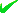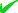News By Tag
Industry News
News By Place

Country(s)

Industry News

May 2023
SuSaFrThWeTuMo
28272625242322

# Near-Field Heat Transfer and Johnson-Nyquist Noise

Extremely low voltage signals of Johnson-Nyquist noise from ambient temperature fluctuations of atoms in surfaces of nanoscale gaps suggest solutions of Maxwell’s equations in near-field heat transfer may not be valid
By: Thomas Prevenslik

Johnson-Nyquist Noise in Nano Gaps
Jan. 11, 2012 - PRLog -- Introduction

Near-field heat transfer by evanescent waves is claimed [1,2] to produce heat fluxes
1000 times greater than  given by Planck’s theory of blackbody  radiation. Based on tunneling of evanescent waves through nanoscale gaps, near-field heat transfer is thought enhanced by exciting dipoles in gap surfaces that are dissipated by Joule heating.  Confirmation of the physics of near-field heat transfer is therefore of great importance in energy harvesting, e.g., the development of high-energy density thermo-photovoltaic devices. See http://www.sciencedaily.com/releases/2009/07/090730154025.htm

Background

In support of near-field heat transfer at the nanoscale, experimental data [1,2] for glass plates is limited by flatness to micron size gaps. At the nanoscale, near-field heat transfer  between microspheres and flat surfaces is promising as flatness problems are avoided. Nevertheless, support for near-field enhancement of heat transfer relies almost entirely on classical solutions by the Maxwell equations, the validity of which has been questioned because by QM the EM confinement of surface atoms precludes the temperature fluctuations necessary to satisfy the FDT. QM stands for quantum mechanics, EM for electromagnetic, and FDT for fluctuation-dissipation theorem. See
See http://www.prlog.org/11759829-invalidity-of-heat-transfer...

Purpose

Invalidity of solutions of Maxwell’s equations in near-field heat transfer because of QM restrictions on the FDT suggests the hope for enhancement in energy harvesting may not be fulfilled. In this regard, a review of the QM restriction on the FDT is presented by considering the voltage signal of the J-N noise from a hypothetical resistor in the surface of a nanoscale gap under EM confinement at ambient temperature. J-N stands for Johnson-Nyquist.

Discussion

In near-field heat transfer solutions of Maxwell equations, the FDT was formulated  based on observations of temperature induced voltage fluctuations of J-N noise in resistors. The J-N noise was derived  for signals at low-frequencies by SM, but the derivation included the QM limitation on the noise signal at high-frequencies that occurs under the EM confinement surface atoms in nanoscale gaps. SM stands for statistical mechanics.

The QM relation for the voltage signals of J-N noise in terms of the EM confinement frequency f is described in the thumbnail. In a gap of dimension d, the EM confinement wavelength w = 2d is related to its frequency f = c/w, where c is the speed of light. R stands for resistance of the resistor, h for Planck’s constant, k for Boltzmann’s constant, and T absolute temperature. The SM relation at high-frequencies is the same as that for QM at low frequencies. For the R = 1000 ohm resistor, variances in J-N noise signal noise are shown for (1) Mean-square voltage^2 / Hertz, and (2) Mean voltage V over the bandwidth B of measurement.

Mean Square Noise. The QM mean square voltage signal was normalized by the SM response. For low-frequencies f < 3x10^11 Hz (d >500 microns), both SM and QM give the same noise signal of 1.65x10^-17 V^2/Hz. SM at high EM confinement frequencies remains the same while the QM signal is reduced. E.g., at f = 3x10^13 Hz (d = 5 microns) the QM signal is 0.0398 x lower, i.e. 6.5x10^-19 V^2/Hz. At f = 3x10^14 Hz (d = 0.5 microns) and 3x10^15 Hz (d = 0.05 microns), the QM noise signal is virtually imperceptible at about 20 and 200 orders of magnitude lower than given by SM.

Noise The noise voltage requires a measurement bandwidth B = (fmax – fmin). Taking fmax = f and fmin = 0, B = f. At low-frequencies f < 3x10^11 Hz (d > 500 microns), both SM and QM give the same noise signal of 2.2 mV.  Again, at higher EM confinement frequencies, the SM noise remains the same while the QM signal is reduced, e.g., at f = 3x10^13 Hz (d= 5 microns) the QM noise is 0.0398 x lower than by SM, i.e. 0.08 mV.  At f = 3x10^14 Hz (d = 0.5 micron) and 3x10^15 Hz (d = 0.05 micron), the QM noise is
imperceptible at about 10 and 100 orders of magnitude lower than given by SM.

It is noted that solutions of Maxwell’s equations assuming the FDT is satisfied require nanoscale gaps d < 0.05 microns to achieve breakthroughs in energy harvesting. But the J-N noise signal at high EM confinement frequencies is extremely weak suggesting the FDT may not be satisfied, and therefore any breakthroughs in energy harvesting may be difficult to achieve in practice.   Further discussion of J-N noise are given in “Johnson-Nyquist Noise,” at http://www.nanoqed.org, 2012.

Conclusions

1. The J-N noise as a measure of the validity of the FDT under EM confinement based on a resistor in the surface of nanoscale gaps having a resistance of 1000 ohms shows the atoms under EM emit almost imperceptible voltage signals at ambient temperature. Hence, the FDT in nanoscale gaps is most likely not satisfied, and therefore near-field heat transfer by the mechanism of tunneling of evanescent waves in nanoscale gaps may not be supported by solutions of Maxwell’s equations suggesting any breakthroughs in energy harvesting may be difficult to achieve in practice.

2. QED induced radiation based on QM is independent of the FDT. EM confinement of thermal emission is precluded in nanoscale gaps, and therefore the surface atoms cannot conserve heat flow by an increase in temperature. Instead, conservation proceeds by the QED induced creation of photons that carry the thermal radiation between gap surfaces.

3. Planck theory is consistent with near-field heat transfer by QED induced radiation.

4. SM that allows the atom to have heat capacity under high EM confinement in nanoscale gaps is refuted by QM.

References

 A. Narayanaswamy et al., “Breakdown of the Planck blackbody radiation law at nanoscale gaps,” Appl Phys A, 96: 357–362 (2009).
  R. S. Ottens, et al., “Near-Field Radiative Heat Transfer between Macroscopic Planar Surfaces,” PRL, 107, 014301 (2011)
  S, M. Rytov, et al,, Principles of Statistical Radiophysics, Springer-Verlag, Berlin, Vol. 3, 1987.
 H. Nyquist, “Thermal Agitation of Electrical Charge in Conductors,” Phys. Rev., 110-113 (1928).

# # #

Classically, absorbed heat from EM sources at the macroscale is conserved by an increase in temperature. But at the nanoscale, temperature increases are forbidden by quantum mechanics. QED radiation explains how heat is conserved by the emission of non-thermal EM radiation to the surroundings.
End
Source Email : Thomas Prevenslik : ***@gmail.com: 15697 : Johnson, Nyquist, Noise, Near Field, Heat Transfer, Quantum Mechanics, Quantum Electrodynamics : Electronics, Energy, Research : Youngwood - Pennsylvania - United States
Account Email AddressAccount Phone NumberDisclaimer     Report Abuse